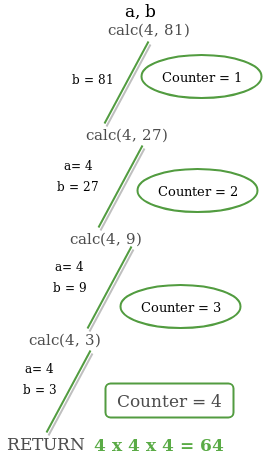Skip to content
Related Articles
GATE | GATE CS 2018 | Question 14
• Last Updated : 23 Feb, 2018

Consider the following C program:

 `#include `` ` `int` `counter = 0;`` ` `int` `calc(``int` `a, ``int` `b) {``  ``int` `c;`` ` `  ``counter++;``  ``if` `(b == 3)``    ``return` `(a * a * a);``  ``else` `{``    ``c = calc(a, b / 3);``    ``return` `(c * c * c);``  ``}``}`` ` `int` `main() {``  ``calc(4, 81);``  ``printf``(``"%d"``, counter);``}`

The output of this program is ________ .

Note – This was Numerical Type question.
(A) 5
(B) 4
(C) 3
(D) None of these

Answer: (B)

Explanation:Quiz of this Question

Attention reader! Don’t stop learning now. Learn all GATE CS concepts with Free Live Classes on our youtube channel.

My Personal Notes arrow_drop_up